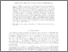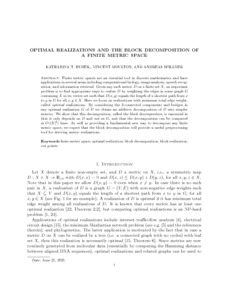# Optimal realizations and the block decomposition of a finite metric space

Huber, Katharina, and Spillner, Andreas (2021) Optimal realizations and the block decomposition of a finite metric space. Discrete Applied Mathematics, 302. pp. 103-113. ISSN 0166-218XPreview PDF (optimal-block-on-pure-2021-06-21) - Accepted Version Available under License Creative Commons Attribution Non-commercial No Derivatives. Download (501kB) | Preview

## Abstract

Finite metric spaces are an essential tool in discrete mathematics and have applications in several areas including computational biology, image analysis, speech recognition, and information retrieval. Given any such metric $$D$$ on a finite set $$X$$, an important problem is to find appropriate~ways to \emph{realize}~$D$ by weighting the edges in some graph~$$G$$ containing $$X$$ in its vertex set such that $$D(x,y)$$ equals the length of a shortest path from $$x$$ to $$y$$ in $$G$$ for all $$x,y \in\nobreak X$$. Here we focus on realizations with minimum total edge weight, called \emph{optimal} realizations. By considering the 2-connected components and bridges in any optimal realization $G$ of $D$ we obtain an additive decomposition of $$D$$ into simpler metrics. We show that this decomposition, called the \emph{block decomposition}, is canonical in that it only depends on $D$ and {\em not} on $G$, and that the decomposition can be computed in $O(|X|^3)$ time. As well as providing a fundamental new way to decompose any finite metric space, we expect that the block decomposition will provide a useful preprocessing tool for deriving metric realizations.

Item Type: Article block decomposition,block realization,cut points,finite metric space,optimal realization,discrete mathematics and combinatorics,applied mathematics ,/dk/atira/pure/subjectarea/asjc/2600/2607 Faculty of Science > School of Computing Sciences http://www.scopus.com/inward/record.url?... LivePure Connector 22 Jun 2021 00:16 20 Apr 2023 21:34 https://ueaeprints.uea.ac.uk/id/eprint/80306 10.1016/j.dam.2021.06.010View Item